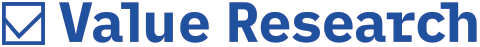# Understanding how risky your fund is

##### Standard deviation is the most common statistical measure of a fund's volatility and riskThe core of fund analysis activity lies in the twin pursuits of judging returns and risk. Stripped of a lot of the complexity, this task involves determining a fund's average performance over a period of time. Let's get down to the basics.

Why do we use an average? Why don't we use the actual numbers that the average is calculated from? Obviously, because an average gives us a single value that represents the numbers it is calculated from. And it is far easier to use a single number for judgement and comparison.

But like most things in life, averages can be both good and bad. They can be trustworthy, or they can be worthless, or they can be anywhere between the two. For example, the average age of children in a kindergarten is three years. You could walk into a kindergarten expecting most kids to be more-or-less three years old and you would not be wrong. However, the average age of students in a school is 12 years. You could walk into a school expecting most students to be more-or-less 12 years old and you would be almost completely wrong. Around 90 per cent of the students would not be 12 years old - they would be all ages from 5 to 18 years (except 12).

What happened? Was the average wrong? Of course not; it just was not a very useful figure. It would have been far more useful if you had been told that most of the individual ages in the kindergarten were close to the average, but most of the individual ages in the school were far from the average. This is exactly what Standard Deviation does. It gives you a 'quality rating' of an average. The Standard Deviation of an average is the amount by which the numbers that go into an average deviate from that average. It tells us how closely an average represents the underlying numbers.

Let's put it this way: There was very little risk in assuming that the kindergarten's average represented the real ages but there was a great deal of risk in assuming that the school's average represented the real ages. Why risk? Isn't that what we are trying to figure out when it comes to our funds? So the recipe for doing that involves these steps:

1. Calculate a fund's monthly performance over a long period of time.
2. Calculate the average of all these monthly performances.
3. If the individual monthly performances is very different from the average, then that fund is risky, delivering high returns in some months and poor returns in others. If they are mostly similar, then the fund is a low risk one, with about the same returns month after month.

And how do we find out if mutual funds are 'very different' or 'mostly similar'? By calculating their average, of course. We first calculate exactly how much each month's performance is different from the average, and then calculate the average of these differences. This is Standard Deviation. While the actual formula is complex, this suffices as an introduction.

But you do need to be careful of one thing - a high Standard Deviation may be a measure of volatility, but it does not necessarily mean that such a fund is worse than one with a low Standard Deviation. If the first fund is a much higher performer than the second one, the deviation will not matter much.